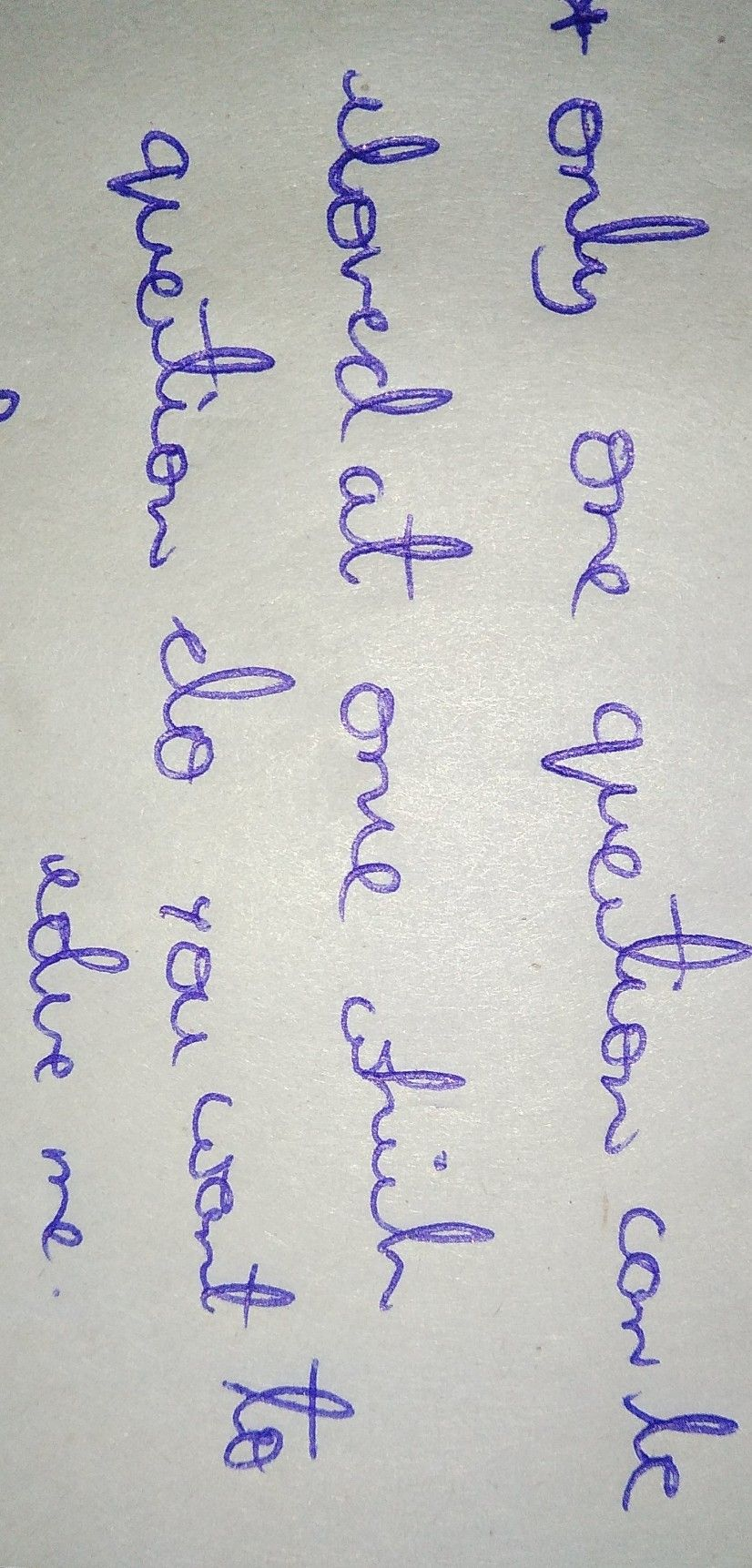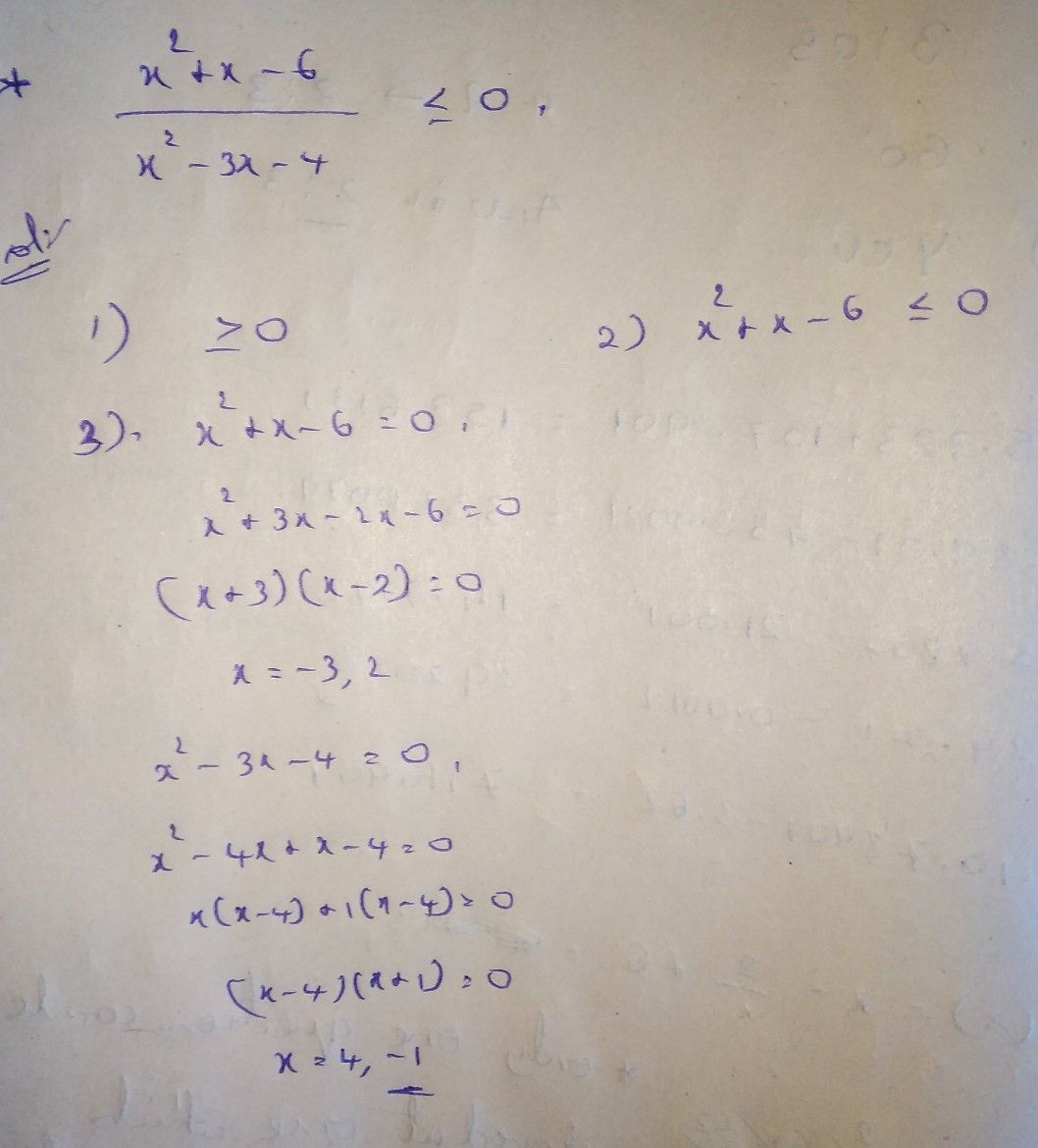Symbol
Problem(You can refer to the review section for solving unlike denominators) 3. Set the equal to numerator and zero and solve. denominator The values 4. you get are called critical values. Plot the critical values on a number line, breaking the number line into intervals. 5. Substitute critical values to the inequality to determine if the endpoints of the intervals in the solution should be included or not. 5. Select test values in each interval SNes. oS6ainotIdlte. ef p ul: nnr t e d e and substitute those values into the inequality. $Note$ If the test value makes the inequality true, then the entire interval is a solution to the inequality. If the test value makes the inequality false, then the entire interval is not a solution to the inequality. 6. Use interval notation or set notation to write the final answer. $0$ $3$ ASea c o 4. Rational Inequality $\dfrac {x^{2}+x-6} {x^{2}-3x-4}\leq 0$ 1. Put the rational inequality in $genera\right)$ form. $\dfrac {R\left(x\right)} {Q\left(x\right)}>0$ where $re>canbe$ replaced by $4$ $and$ 2 $2.1$ Write the inequality into a single rational expression on the left side. (You can refer to the review section for solving unlike denominators) $3.9$ Set the numerator and denominator equal to zero and solve. The values you get are called critical values. $4.$ $Plot$ the critical values on a number line, breaking the number line into intervals. $5.S$ Substitute critical values to the inequality to determine if the $8$
10th-13th grade
Geometry
Search count: 140
SolutionQanda teacher - Aravind